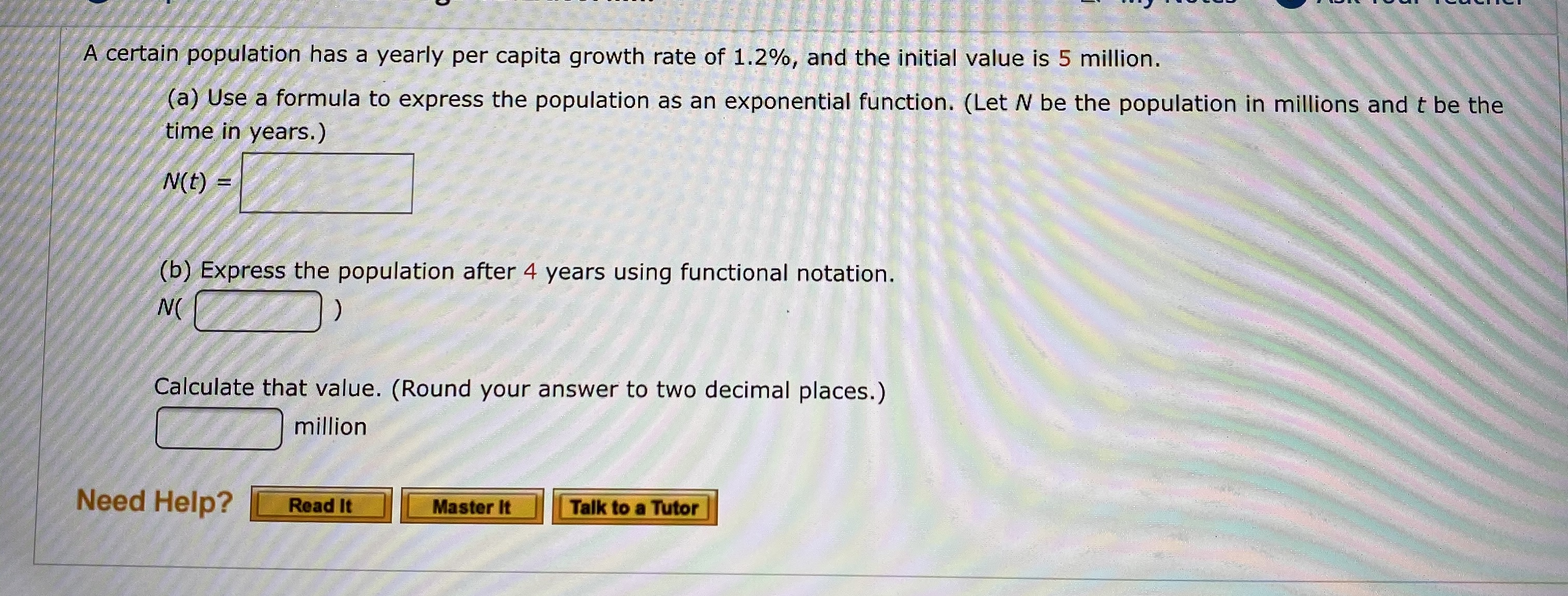# A certain population has a yearly per capita growth rate of 1.2%, and the initial value is 5 million.(a) Use a formula to express the population as an exponential function. (Let N be the population in millions and t be thetime in years.)N(t)(b) Express the population after 4 years using functional notation.N(Calculate that value. (Round your answer to two decimal places.)millionNeed Help?Read ItTalk to a TutorMaster It

Question
1 views

What are the blanks?help_outlineImage TranscriptioncloseA certain population has a yearly per capita growth rate of 1.2%, and the initial value is 5 million. (a) Use a formula to express the population as an exponential function. (Let N be the population in millions and t be the time in years.) N(t) (b) Express the population after 4 years using functional notation. N( Calculate that value. (Round your answer to two decimal places.) million Need Help? Read It Talk to a Tutor Master It fullscreen
check_circle

Step 1

To identify the gr...

### Want to see the full answer?

See Solution

#### Want to see this answer and more?

Solutions are written by subject experts who are available 24/7. Questions are typically answered within 1 hour.*

See Solution
*Response times may vary by subject and question.
Tagged in

### Algebra